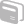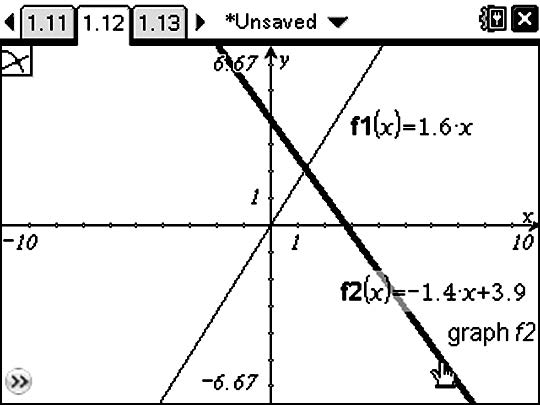# Activities

••• ##### Subject Area

• Math: Algebra I: Systems of Linear Equations

• ##### Author9-12

45 Minutes

• ##### Device
•TI-Nspire™ CX/CX II
•TI-Nspire™ CX CAS/CX II CAS
• TI-Nspire™
• TI-Nspire™ CAS
• ##### Software

TI-Nspire™
TI-Nspire™ CAS

3.0

## Solving a Pair of Linear Equations by Graphing#### Activity Overview

Explore moving a point to illustrate solving systems of linear equalities graphically.

#### Objectives

• Graph a pair of linear equations and estimate their solution using the Intersection Point(s) command
• Verify the solution of a linear system by substitution of the solution into both equations